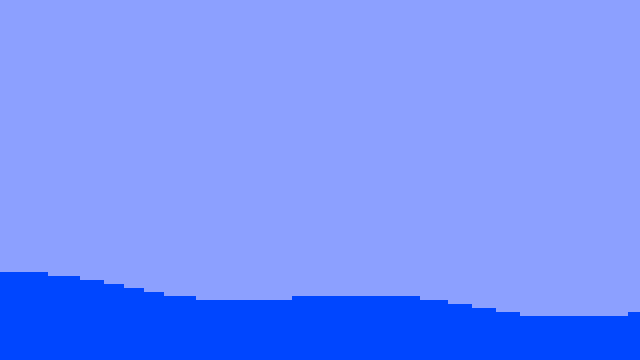# Small programs

Some small C programs with big consequences.
Let's pretend it's art.

## Mandelbrot

The following program generates the following image.

```#include<stdio.h>
double X=-1.256,Y=.38,R=.02;
int c;for(c=255;c>0;c--){double x1=x*x-y*y+d,y1=2*x*y+e,r=x1*x1+y1*y1;if(r>4)
break;x=x1;y=y1;}return c;}int main(void){int i,j;for(j=0;j<768;j++)for(i=0;i
<1024;i++){unsigned char x=a(i,j);if(x>232)x=0;if(x<128)x=0;else if(x>200)x=(
256-x)*2;else x=x-100;putchar(x);}fflush(stdout);return 0;}
```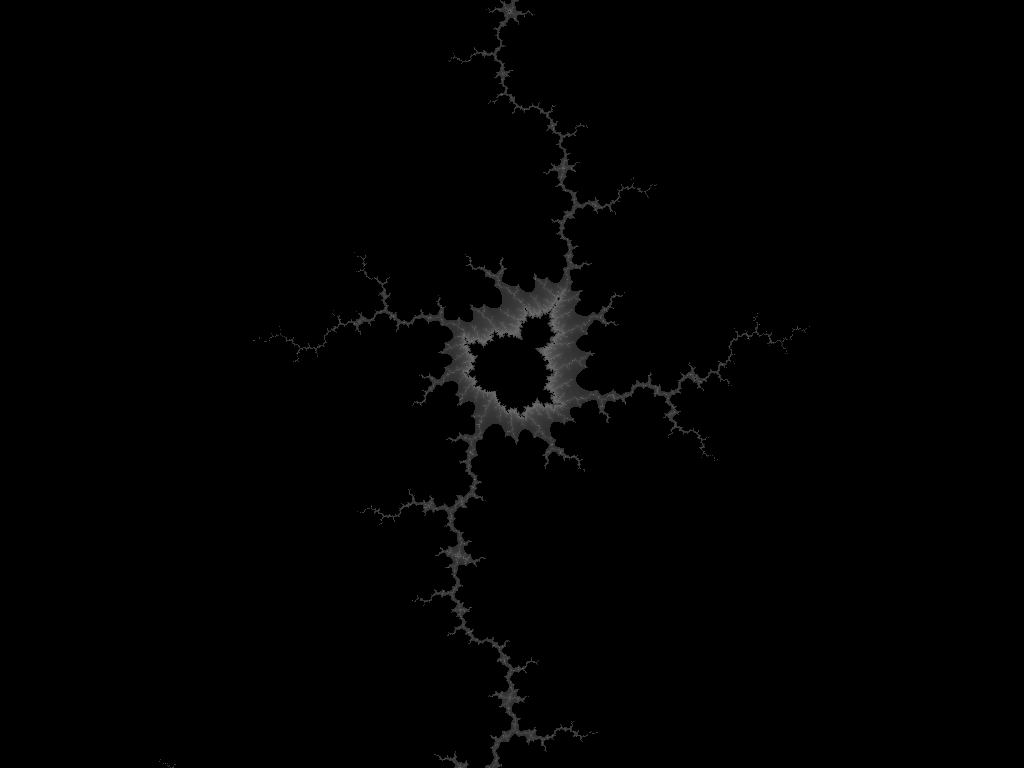This is the most famous example of fractals.

## Mandelbrot zoom

A small variation of the previous program, generating a video.

```#include<stdio.h>
#include<math.h>
double X,Y,R,o,i,j,k,z,d,e,x,y,t,u,r;int main(void){for(;k<=1.005;k+=.005){X=-
.5+k*-0.756;Y=k*.38;R=2+k*-1.98;o=k*6.18;for(j=0;j<240;j++)for(i=0;i<320;i++){
x=i*R/120+X-R*4/3;y=j*-R/120+Y+R;d=(x-X)*cos(o)-(y-Y)*sin(o)+X;e=(x-X)*sin(o)+
(y-Y)*cos(o)+Y;x=d;y=e;r=0;for(z=255;z>0&&r<=4;z--){t=x*x-y*y+d,u=2*x*y+e,r=t*
t+u*u;x=t;y=u;}z=z>232||z<128?0:z>200?(256-z)*2:z-100;putchar(z);}}fflush(
stdout);return 0;}
```

To create the video, I ran:

```ffmpeg -f rawvideo -s 320x240 -r 25 -pix_fmt gray -i /tmp/A -vf scale=1024:768 -sws_flags neighbor -b:v 1M /tmp/A.ogg
```

I love the pixelated look (even if the encoding destroys it a little bit, not squared enough).

## Sierpinski

This one I found by accident while having fun with assembly language and good old mode 13h (old memories). I was doing plot(x, x and y) by mistake, and that was the result. Here we use a different but equivalent trick. The reader will have to figure out by herself, or politely ask me and I'll be more than happy to explain. I still remember how surprized (surprised?) I was when that Sierpinski's triangle popped up on my dark screen, late at night.

I embellished a bit to create a video (animated GIF actually, to annoy you, even though I don't know who you are and I doubt I would want to annoy you in the first place so let me apologize for this) instead of a single image.

```#include <stdio.h>
#include <math.h>
int main(void){double B,C,D,E;int A,i,j,u,v;for(A=0;A<240;A++){B=M_PI*2/
(240./3)*A;C=sin(B)*.5+1;for(j=0;j<240;j++)for(i=0;i<320;i++){D=160+cos(
B/3)*80;E=120+sin(B/3)*80;u=50+C*(cos(B)*(i-D)-sin(B)*(j-E));v=80+C*(sin
(B)*(i-D)+cos(B)*(j-E));putchar(-((u&v)==u));}}}
```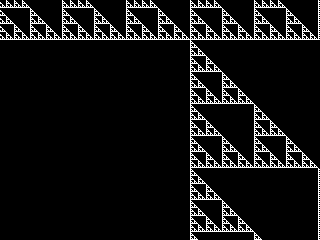To produce the video, I ran:

```gcc -Wall sierpinski.c  -lm -O3; ./a.out > KA; ffmpeg -f rawvideo -s 320x240 -r 24 -pix_fmt gray -i KA sierpinski.gif
```

## Eclipse

I was inspired by the total solar eclipse we've had 1999-08-11 not too far from Paris. Some friend of mine (Hulud, if you read this...) had a car and we went to Étretat to experience it fully. All in a sudden all was dark and cold. Everyone shouted. Happy? Then the light and warmth came back. Strange and good moment.

Technical details on demand. It's just a bunch of tricks in there, nothing really fancy. You may also see this, the effect is in there with a more expansed source code (maybe not enough to understand though).

This program is a bit long, sorry for that.

```#include<math.h>
#include<unistd.h>
#include<stdlib.h>
#define F(Y,Z)for(Y=0;Y<Z;Y++)
#define R rand()/(RAND_MAX+1.)
unsigned char A,B,C,D,*p,*t;int E,
i,j,k,z,X,Y,d,g,a,b;double l,x,y,m;int main(void){F(i,32)F(j,32){k=(14-sqrt((
16-i)*(16-i)+(16-j)*(16-j)))*255/14;if(k>0)B[i][j]=k;}F(i,256)F(j,256)C[i][j]=
i*j/255,D[i][j]=i+j>255?255:i+j;F(i,100){x=i/99.*319;l=x-160;l*=l;F(j,100){y=j
/99.*239;m=y-120;m*=m;if(l+m<2924){E[z]=E[z+1182]=x;E[z++]=E[z+1182][
1]=y;}}}F(l,250){F(i,76800)A[i]=0;x=l*1.68-50;y=290-l*1.36;g=R*z;a=R*10-5;b=R*
10-5;F(k,z){X=E[k+g]-x;Y=E[k+g]-y;d=X*X+Y*Y-2209;if(d>0){d=sqrt(d);if(d>
255)d=255;p=&B;if(d>19){t=A+E[k+g]+a-16+(E[k+g]+b-16)*320;if(t[
5136]<81){F(i,32){F(j,32)*t=D[*t][C[d][*p]],p++,t++;t+=288;}}}}}write(1,A,
76800);}}
```

## Spirals

[2020-12-29]

This is my version to generate this (sorry if deadlink, this is out of my control).

The code is not very small. And not obfuscated. But it's still quite small. Minification and obfuscation left as an exercise to the reader! (Send me your work!)

spirals.c contains the code.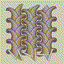(Click this small image for a larger version. The file is big though!)

And here is the order of plotting all the bars. Let's reveal the trick!If you edit the code, look for this line:

```    if (repaint) { paint(curtime, 0, 0); redraw = 1; repaint = 0; }
```

And replace by this line:

```    if (repaint) { paint(curtime, 0, 1); redraw = 1; repaint = 0; }
```

Then you also get the plotting done bar by bar in X Window mode.

Oh yes, only works on X Window. Is there something else out there?

Ah, you can comment out the X Window code and have it compile on other systems. (Exercise two for the reader, way easier than minification and obfuscation.) You still need cairo for the plotting (which you want to get rid of for the minification I'd say, and find a small algorithm to plot the bars).

The program is very slow, sorry for that. Let's pretend cairo is the guilty one.

I think it should not be too hard to write a javascript/canvas version. Exercise three for the reader. Send me your work!

## Hyperbole

[2021-02-27]

```#include<stdio.h>
#include<math.h>
char A[1<<18];int main(void){double K=0.5257311121191334,J=0.8506508083520399,
B,C,D,E,F,G,H;int I,L;for(B=0;B<60;B++){for(I=0;I<1<<18;)A[I++]=0;for(C=-2;C<2
;C+=.2)for(D=-2;D<2;D+=.2){E=exp(-0.016040394168653451*B+0.24060591252980173);
G=E*1.6180339887498949*C-E*D;H=0.618033988749894903/E*C+D/E;E=J*G-K*H;F=K*G+J*
H;G=E*256+256;H=511-(F*256+256);for(I=G-32;I<G+32;I++)for(L=H-32;L<H+32;L++)if
(!(I&~511)&!(L&~511)&&(I-G)*(I-G)+(L-H)*(L-H)<=1<<10)A[I*512+L]=128;}fwrite(A,
1<<18,1,stdout);}return 0;}
```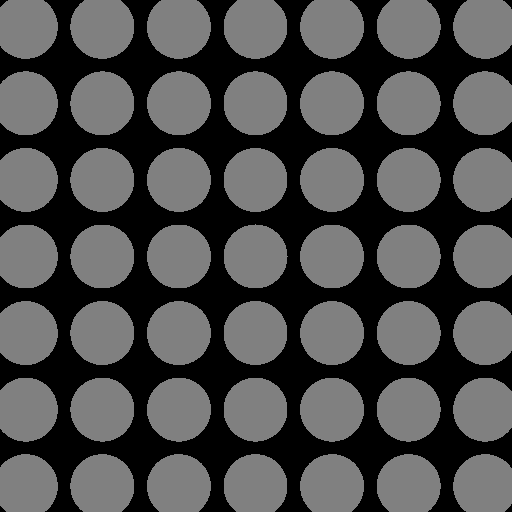## 10order

[2021-02-27]

(10 is pronounced "dis" in french.)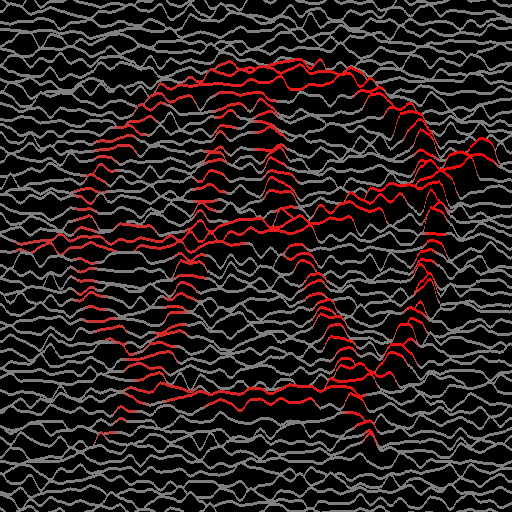Was nice to do, lots of fun. I should describe the various tricks involved.

Maybe I'll do an obfuscated version when I feel like I want to (but if I wait too much then when I read the code I won't understand it anymore...). Bah, so be it. Laziness! I love you.

## Pi day

Pi is special. It has its own webpage. And with some javascript in it!

## p-norm

[2022-09-07]

P-norm is funny. Let's have fun with it.

norm.c generates an animation. Here are a few screenshots.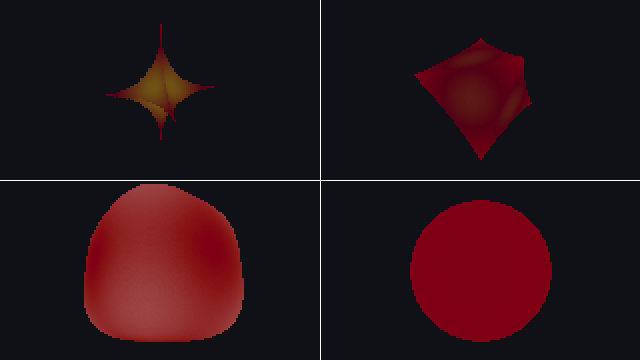The animation is norm.gif, big file!

And norm_infinity.c does another animation, with more stuff. Some screenshots? There you go.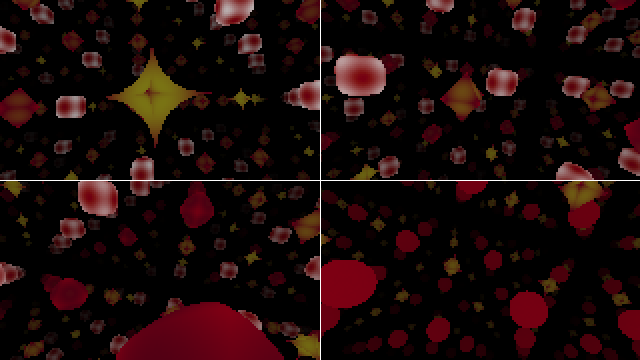And there comes norm_infinity.gif!

I loved doing this! My only problem is that these programs are not realtime. I've always been bad with raytracing... I'm almost sure this can be rendered in realtime. If you read this and can help...

2022-10-07: I made some GLSL versions of the above! Check here. Might be realtime (that is: more than 25 frames per second) depending on your GPU. This GPU thing is insane me says.

## koch

[2022-11-04]

Let's have fun with the Koch curve.

koch.c produces the following image when piped with gui.c and then some ffmpeg magic to generate the GIF.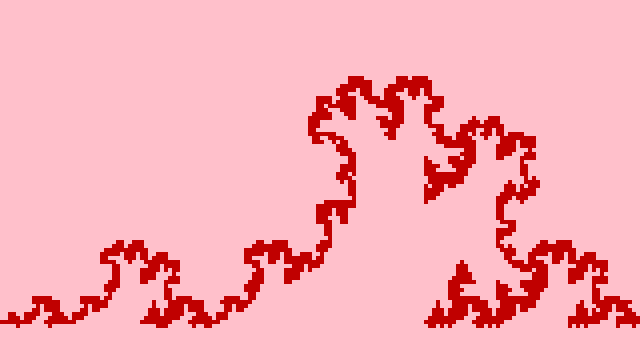koch-full.c is a little variation. I struggled a bit for this one! I'm not proud...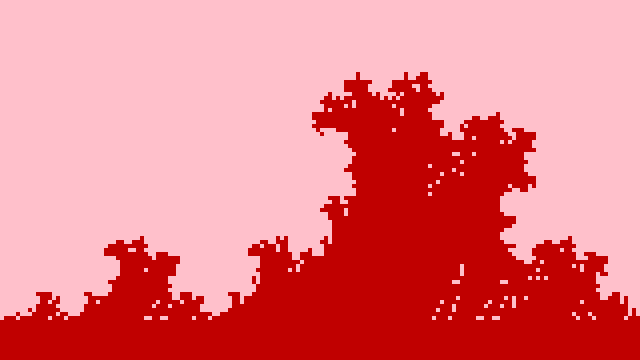I think I prefer first version. I'm not sure.